# 大数据画像丨如何进行用户数据挖掘？

2019-05-21来源：五服茶馆

1.朴素贝叶斯的原理

P(A|B)表示事件B已经发生的前提下，事件A发生的概率，叫做事件B发生下事件A的条件概率。其基本求解公式为：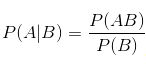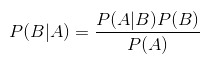2.朴素贝叶斯的数据挖掘原理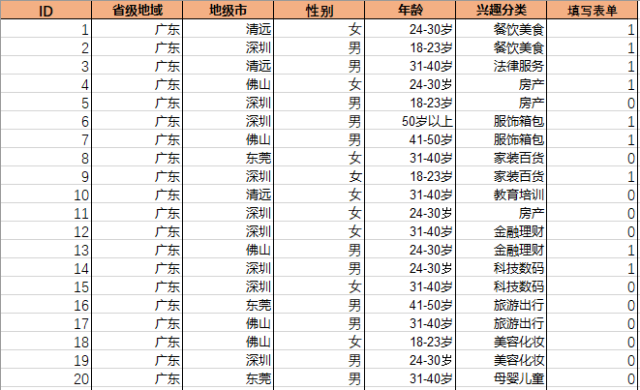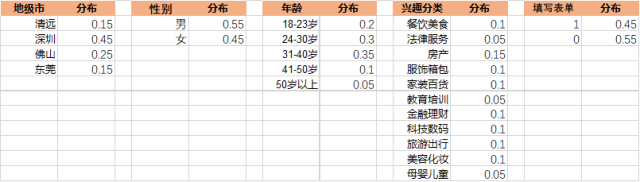P（填写表单="1" | X) = P（X | 填写表单="1") * P（填写表单="1") / P（X）

P（填写表单="0" | X) = P（X | 填写表单="0") * P（填写表单="0") / P（X）

P（填写表单="1" | X) = P（X | 填写表单="1") * P（填写表单="1") / P（X）= （3/9 * 6/9 * 2/9 * 1/9）*0.45 / P（X） = 0.002469 / P（X）...................................................①

P（填写表单="0" | X) = P（X | 填写表单="0") * P（填写表单="0") / P（X）= （2/11 * 5/11 * 2/11 * 2/11）*0.55 / P（X） = 0.0015026 / P（X）...................................................②

P（填写表单="1" | X) + P（填写表单="0" | X) =1...................................................③

P（填写表单="1" | X) = 62.2%

P（填写表单="0" | X) = 37.8%

3.朴素贝叶斯的数据挖掘的优势

4.朴叶斯的数据挖掘案例解读

4.1 原生数据及预处理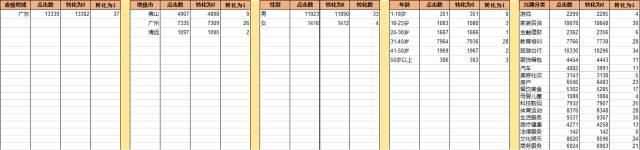4.2 计算字段重要性，确定输入字段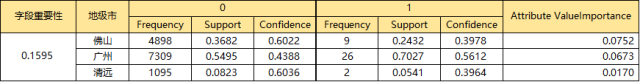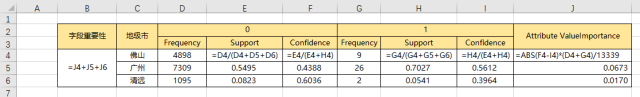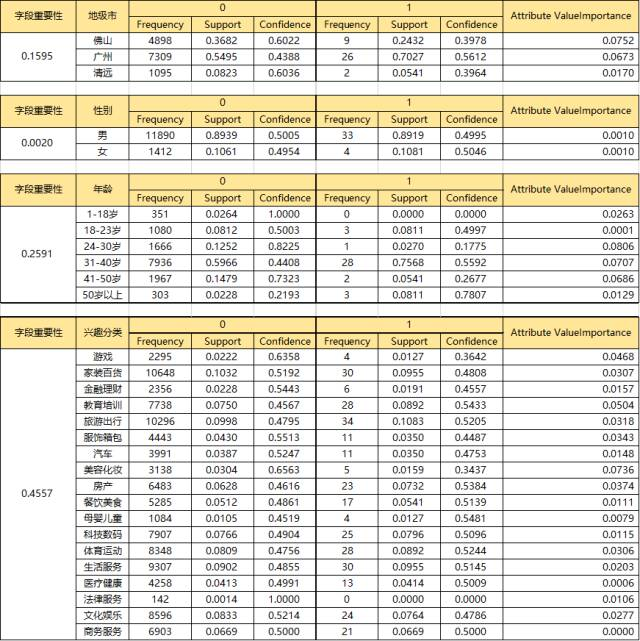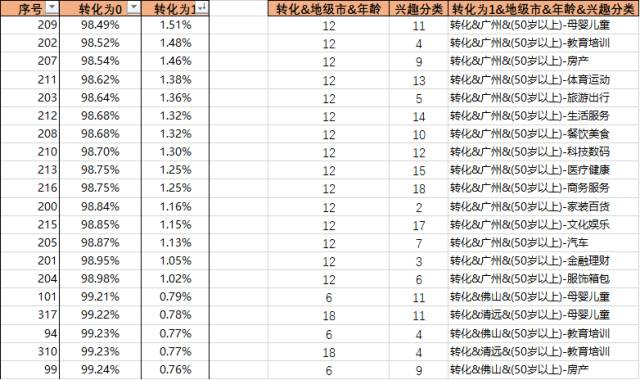。。。（中间太长，省略了）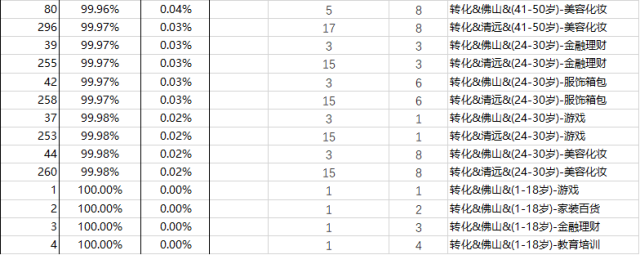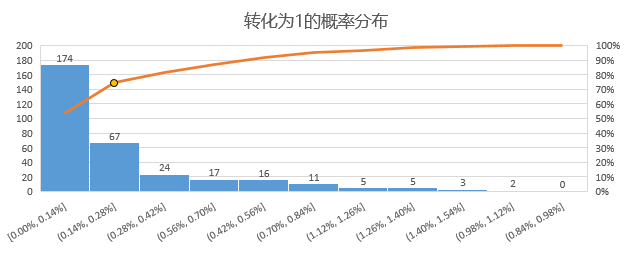【实时分析型数据库】